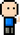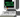# Josh Meets ComputerApril 13, 2018

# Graph Search

Algorithms for finding paths, searching for nodes in unweighted and weighted graphs

``````from IPython.display import display, HTML

CSS = """
.output {
margin: left;
flex-direction: row;
border-style: dotted;
}
"""
HTML('<style>{}</style>'.format(CSS))``````

## Shortest Path Algorithm

Find shortest path to all other nodes from given node

``````def all_shortest_paths(G, source):
assert source in G, 'Source node not in Graph'

this_level = [source]
paths_to = {source: [source]}

next_level = first_level

while next_level:
curr_level = next_level
next_level = []

for curr_node in curr_level:
if next_node not in paths_to:
paths_to[next_node] = paths_to[curr_node] + [next_node]
next_level += [next_node]

return paths_to``````
``````import networkx as nx
import matplotlib.pyplot as plt

G = nx.DiGraph()

plt.figure(figsize=(6,4))
plt.axis('off')
for target, path in all_shortest_paths(G, 'C').items():
print('From C to {}: \n\t{}'.format(target, '->'.join(path)))

nx.draw_networkx(G)
plt.show()``````
``````From C to F:
C->D->E->F
From C to E:
C->D->E
From C to C:
C
From C to D:
C->D
From C to G:
C->D->E->G``````